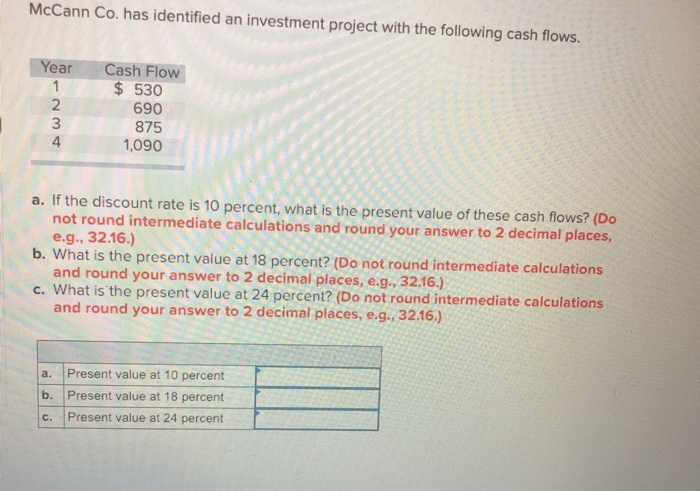# Mccann co. has identified an investment project with the following cash flows.McCann Co. has identified an investment project with the following cash flows. Year Cash Flow 2 3 4 530 690 875 1090 a. If the discount rate is 10 percent, what is the present value of these cash flows? (Do not round intermediate calculations and round your answer to 2 decimal places, e.g.32.16.) and round your answer to 2 decimal places, e.g., 32.16.) and round your answer to 2 decimal places, e.g., 32.16.) b. What is the present value at 18 percent? (Do not round intermediate calculations c. What is the present value at 24 percent? (Do not round intermediate calculations a. Present value at 10 percent b. Present value at 18 percent c. Present value at 24 percent

Present value of cash flows = CF1/(1+r)^1 + CF2/(1+r)^2
…………CFn/(1+r)^n
a:
At 10%
PV = 530/1.1^1 + 690/1.1^2+ 875/1.1^3 + 1090/1.1^4
=2453.95
b:
At 18%
PV = 530/1.18^1 + 690/1.18^2+ 875/1.18^3 + 1090/1.18^4
=2039.46
c:
At 24%
PV = 530/1.24^1 + 690/1.24^2+ 875/1.24^3 + 1090/1.24^4
=1796.14